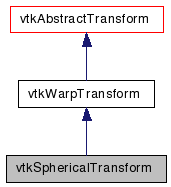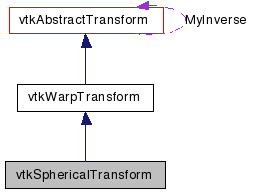# vtkSphericalTransform Class Reference

`#include <vtkSphericalTransform.h>`

Inheritance diagram for vtkSphericalTransform:[legend]
Collaboration diagram for vtkSphericalTransform:[legend]

## Detailed Description

spherical to rectangular coords and back

vtkSphericalTransform will convert (r,phi,theta) coordinates to (x,y,z) coordinates and back again. The angles are given in radians. By default, it converts spherical coordinates to rectangular, but GetInverse() returns a transform that will do the opposite. The equation that is used is x = r*sin(phi)*cos(theta), y = r*sin(phi)*sin(theta), z = r*cos(phi).

Warning:
This transform is not well behaved along the line x=y=0 (i.e. along the z-axis)
vtkCylindricalTransform vtkGeneralTransform
Examples:
vtkSphericalTransform (Examples)
Tests:
vtkSphericalTransform (Tests)

Definition at line 42 of file vtkSphericalTransform.h.

## Public Types

typedef vtkWarpTransform Superclass

## Public Member Functions

virtual const char * GetClassName ()
virtual int IsA (const char *type)
virtual void PrintSelf (ostream &os, vtkIndent indent)
vtkAbstractTransformMakeTransform ()

## Static Public Member Functions

static vtkSphericalTransformNew ()
static int IsTypeOf (const char *type)
static vtkSphericalTransformSafeDownCast (vtkObject *o)

## Protected Member Functions

vtkSphericalTransform ()
~vtkSphericalTransform ()
void InternalDeepCopy (vtkAbstractTransform *transform)
void ForwardTransformDerivative (const float in, float out, float derivative)
void ForwardTransformDerivative (const double in, double out, double derivative)
void InverseTransformPoint (const float in, float out)
void InverseTransformPoint (const double in, double out)
void InverseTransformDerivative (const float in, float out, float derivative)
void InverseTransformDerivative (const double in, double out, double derivative)
void ForwardTransformPoint (const float in, float out)
void ForwardTransformPoint (const double in, double out)

## Member Typedef Documentation

Reimplemented from vtkWarpTransform.

Definition at line 46 of file vtkSphericalTransform.h.

## Constructor & Destructor Documentation

 vtkSphericalTransform::vtkSphericalTransform ( ) ` [protected]`

 vtkSphericalTransform::~vtkSphericalTransform ( ) ` [protected]`

## Member Function Documentation

 static vtkSphericalTransform* vtkSphericalTransform::New ( ) ` [static]`

Create an object with Debug turned off, modified time initialized to zero, and reference counting on.

Reimplemented from vtkObject.

 virtual const char* vtkSphericalTransform::GetClassName ( ) ` [virtual]`

Reimplemented from vtkWarpTransform.

 static int vtkSphericalTransform::IsTypeOf ( const char * name ) ` [static]`

Return 1 if this class type is the same type of (or a subclass of) the named class. Returns 0 otherwise. This method works in combination with vtkTypeMacro found in vtkSetGet.h.

Reimplemented from vtkWarpTransform.

 virtual int vtkSphericalTransform::IsA ( const char * name ) ` [virtual]`

Return 1 if this class is the same type of (or a subclass of) the named class. Returns 0 otherwise. This method works in combination with vtkTypeMacro found in vtkSetGet.h.

Reimplemented from vtkWarpTransform.

 static vtkSphericalTransform* vtkSphericalTransform::SafeDownCast ( vtkObject * o ) ` [static]`

Reimplemented from vtkWarpTransform.

 virtual void vtkSphericalTransform::PrintSelf ( ostream & os, vtkIndent indent ) ` [virtual]`

Methods invoked by print to print information about the object including superclasses. Typically not called by the user (use Print() instead) but used in the hierarchical print process to combine the output of several classes.

Reimplemented from vtkWarpTransform.

 vtkAbstractTransform* vtkSphericalTransform::MakeTransform ( ) ` [virtual]`

Make another transform of the same type.

Implements vtkAbstractTransform.

 void vtkSphericalTransform::InternalDeepCopy ( vtkAbstractTransform * transform ) ` [protected, virtual]`

Copy this transform from another of the same type.

Reimplemented from vtkAbstractTransform.

 void vtkSphericalTransform::ForwardTransformPoint ( const float in, float out ) ` [protected, virtual]`

Internal functions for calculating the transformation.

Implements vtkWarpTransform.

 void vtkSphericalTransform::ForwardTransformPoint ( const double in, double out ) ` [protected, virtual]`

Internal functions for calculating the transformation.

Implements vtkWarpTransform.

 void vtkSphericalTransform::ForwardTransformDerivative ( const float in, float out, float derivative ) ` [protected, virtual]`

Calculate the forward transform as well as the derivative.

Implements vtkWarpTransform.

 void vtkSphericalTransform::ForwardTransformDerivative ( const double in, double out, double derivative ) ` [protected, virtual]`

Calculate the forward transform as well as the derivative.

Implements vtkWarpTransform.

 void vtkSphericalTransform::InverseTransformPoint ( const float in, float out ) ` [protected, virtual]`

If the InverseFlag is set to 1, then a call to InternalTransformPoint results in a call to InverseTransformPoint. The inverse transformation is calculated from using Newton's method.

Reimplemented from vtkWarpTransform.

 void vtkSphericalTransform::InverseTransformPoint ( const double in, double out ) ` [protected, virtual]`

If the InverseFlag is set to 1, then a call to InternalTransformPoint results in a call to InverseTransformPoint. The inverse transformation is calculated from using Newton's method.

Reimplemented from vtkWarpTransform.

 void vtkSphericalTransform::InverseTransformDerivative ( const float in, float out, float derivative ) ` [protected, virtual]`

Calculate the inverse transform as well as the derivative of the forward transform (that's correct: the derivative of the forward transform, not of the inverse transform)

Reimplemented from vtkWarpTransform.

 void vtkSphericalTransform::InverseTransformDerivative ( const double in, double out, double derivative ) ` [protected, virtual]`

Calculate the inverse transform as well as the derivative of the forward transform (that's correct: the derivative of the forward transform, not of the inverse transform)

Reimplemented from vtkWarpTransform.

The documentation for this class was generated from the following file:

Generated on Wed Aug 24 12:07:46 2011 for VTK by1.5.6